# Subtraction Action! Ordering Numbers

With the help of these colorful critters, your second grader will practise double-digit subtraction AndOrdering numbers from least to greatest.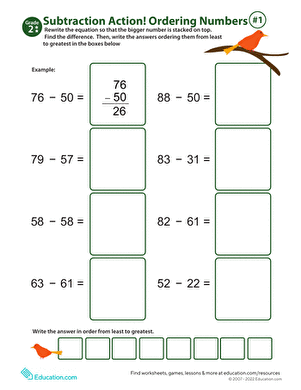### Subtraction Action! Ordering Numbers #1

Maximize your second grader's at-home maths practise with this fun worksheet, which combines subtraction and number ordering.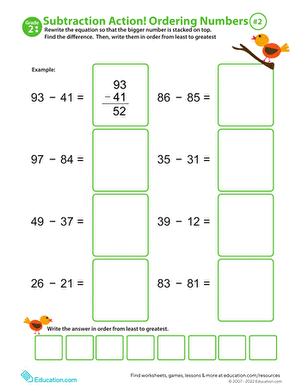### Subtraction Action! Ordering Numbers #2

Incorporate both double-digit subtraction and ordering numbers into just one colorful worksheet!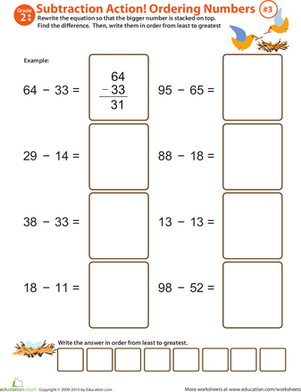### Subtraction Action! Ordering Numbers #3

This colorful maths worksheet has two advantages for your second grader! She'll boost her subtraction skills as she practices ordering numbers.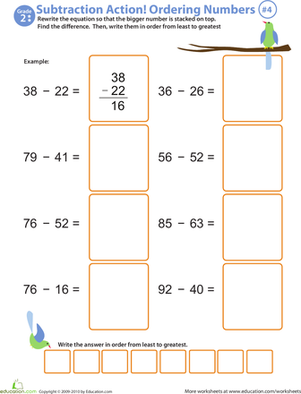### Subtraction Action! Ordering Numbers #4

This worksheet is a double whammy; your second grader will practise double-digit subtraction and work on ordering numbers from least to greatest.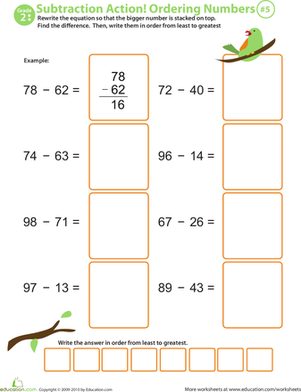### Ordering Numbers

Let the pretty bird perched at the top of this worksheet be your second grader's guide through double-digit subtraction and ordering numbers.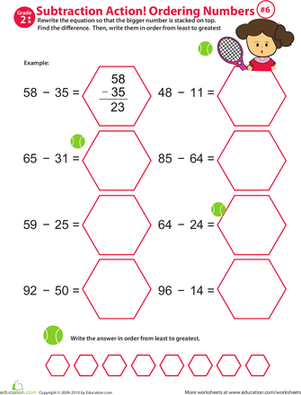### Subtraction Action! Ordering Numbers #6

Your second grader will be challenged to solve double-digit subtraction and practise ordering numbers in this tennis-themed worksheet!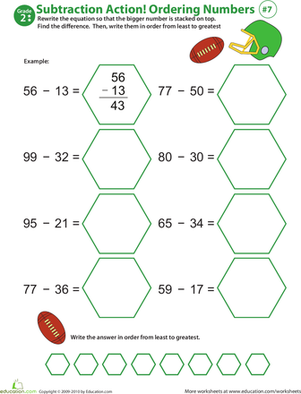### Subtraction Action! Ordering Numbers #7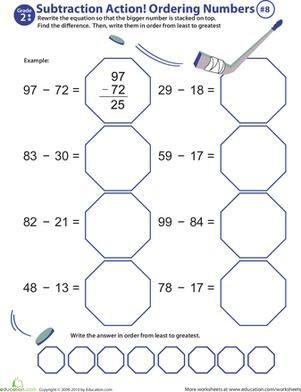### Subtraction Action! Ordering Numbers #8

This colorful worksheet will give your second grader the boost she needs to become a subtraction know-it-all!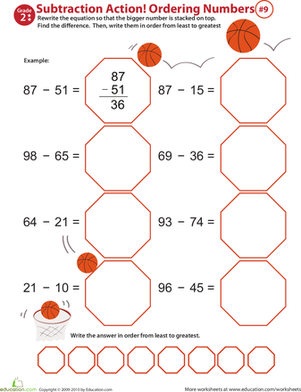### Subtraction Action! Ordering Numbers #9

Spark your second grader's interest in subtraction with this fun, colorful worksheet!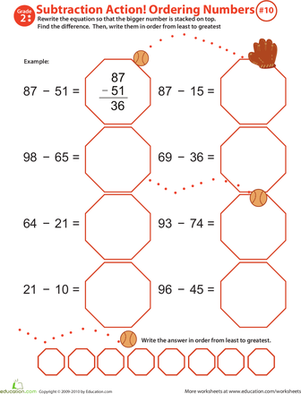### Subtraction Action! Ordering Numbers #10

This delightful worksheet will maximize your second grader's at-home maths time. He'll learn subtraction and practise ordering numbers from least to greatest.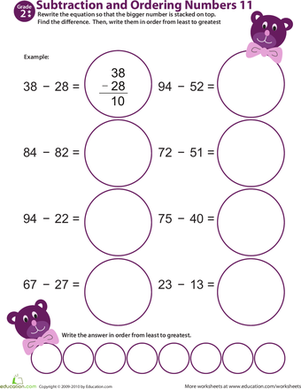### Subtraction Action! Ordering Numbers #11

Get your child excited about subtraction with this year 3 maths worksheet in which she'll practising writing equations, subtracting, and ordering numbers.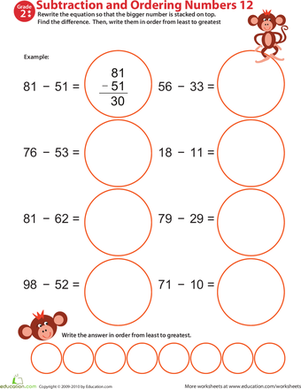### Subtraction Action! Ordering Numbers #12

More than your average year 3 maths worksheet, this printable makes practising two-digit subtraction and place value fun!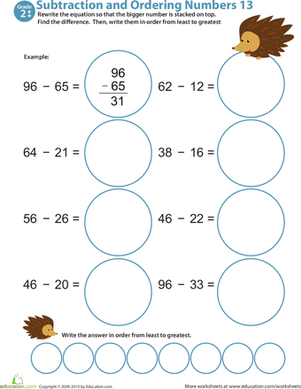### Subtraction Action! Ordering Numbers #13

Help your child improve his subtraction facts fluency with this year 3 maths worksheets featuring two-digit subtraction and number ordering.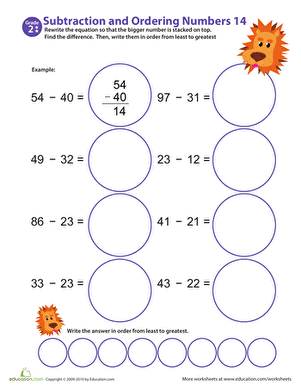### Subtraction Action! Ordering Numbers #14

Help your child master two-digit subtraction with this cheerful maths worksheet in which he'll rewrite equations, solve, then put his answers in numerical order.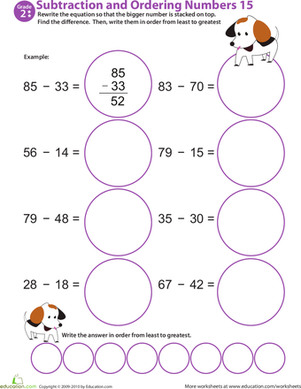### Subtraction Action! Ordering Numbers #15

Download this year 3 maths worksheet to give your child valuable at-home subtraction practise. Offers two-digit subtraction and a number ordering exercise.

Create new collection

0

### New Collection>

0Items

What could we do to improve Education.com?